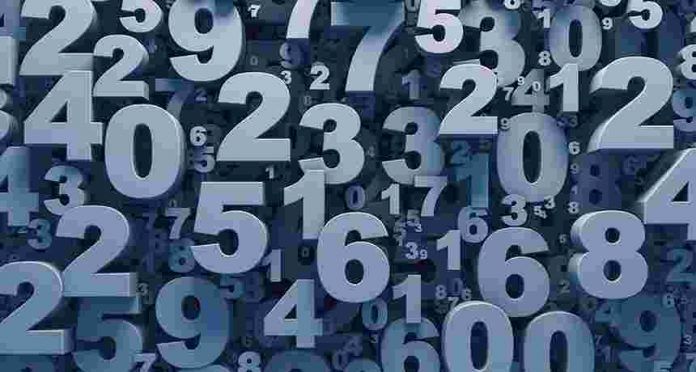# Numerology indian name calculatorHere we have numerology calculator for Indian names and other names by date of birth. Basically, numerology is related to the numbers. Each letter in the English alphabet is equal to some number. The letters in our name will be matched with those numbers to predict the future.

Numerology calculator

Name Date of birth

Here we have provided Life path number, Destiny number, personality number and soul urge number based on your name and date of birth.
Now we will see how to calculate all those numerology numbers by name and date of birth(DOB).

Life path Number calculation in Numerology

Life path numerology number will be calculated based on the date of birth. Consider your birthdate is April 5, 1998. Now we need to split all these numbers into a single digit and need to sum it up.

- Advertisement -

April is the 4th month. So the number for it will be 4.
4 + 5 + 1 + 9 + 9 + 8 = 36

If the result is a double-digit number(i.e greater than or equal to 10) then again we need to split it. Numerology number will always be single digit so we need to continuously split it and sum it up until we get a single digit.

36 = 3 + 6 = 9

That’s it. 9 is the life path number of a person who born on April 5, 1998.

Destiny Number Calculation in Numerology

Destiny numerology number will be calculated based on the letters in your name. Now we will see one table which contains the numerology number corresponding to the letters in English albhabets.

Numerology number table

LetterNumber
A, J, S1
B, K, T2
C, L, U3
D, M, V4
E, N, W5
F, O, X6
G, P, Y7
H, Q, Z8
I, R9

Now let’s take the name Mike and calculate a destiny numerology number for that name.
From the above table, 1st we need to assign the number for corresponding letters in the name.

M = 4
I = 9
K = 2
E = 5

Now we need to sum up all those numbers.
4 + 9 + 2 + 5 = 20
If the resultant number is two digit then we need to split it and again we need to sum up until we get a single digit.
20 = 2 + 0 = 2

So for the name Mike, the destiny numerology number will be 2.

Personality number calculation in Numerology

Personality numerology number also be calculated based on the letters in our name. But here we need to take only consonants in our name.

Now we will take the same example, Mike. In this name, I and E are not consonants. So we need to avoid it and consider the rest of the letters to calculate the personality number.

M = 4
K = 2
(Check the above numerology number table to get the value for each letter)

Now we need to sum up these and need to split it until we get a single digit.
4 + 2 = 6

So the personality numerology number for the name Mike is 6.

Soul Urge Number calculation in Numerology

To calculate the soul urge number we need to consider only vowels in our name. Now, let’s take the same example, Mike. In this name I and E are vowels. So we need to consider only these two letters to calculate the soul urge number.

I = 9
E = 5
(Check the above numerology number table to get the value for each letter)

Now we need to sum up these numbers first. If it is double digit then we need to and split it and sum up. we need to repeat this operation until we get a single digit.

9 + 5 = 14
1 + 4 = 5

So the soul urge numerology number for the name Mike is 5